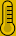# Products - Ensapsultated transformers searcher

Search by power

Power Primary Secondary type Secondary voltageSearch by current

Primary Secondary type Secondary voltage

Intensity s1+s2

In miliampers

You can also request a custom transformer

{if \$type_search eq 1 or \$type_search eq 2}

{if \$quantity neq 0} Results

{section name=i loop=\$found} {/section}
HR Ref. Power Primary 1 Primary 2 Secondary 1 Secondary 2{\$found[i].ref} {\$found[i].va} VA {if \$found[i].p1 eq 0} - {else} {\$found[i].p1} V {/if} {if \$found[i].p2 eq 0} - {else} {\$found[i].p2} V {/if} {if \$found[i].s1 eq 0} - {else} {\$found[i].s1} V {/if} {if \$found[i].s2 eq 0} - {else} {\$found[i].s2} V {/if} {\$found[i].temperature}ºC/B
{else} Not matches found

{/if} {/if}